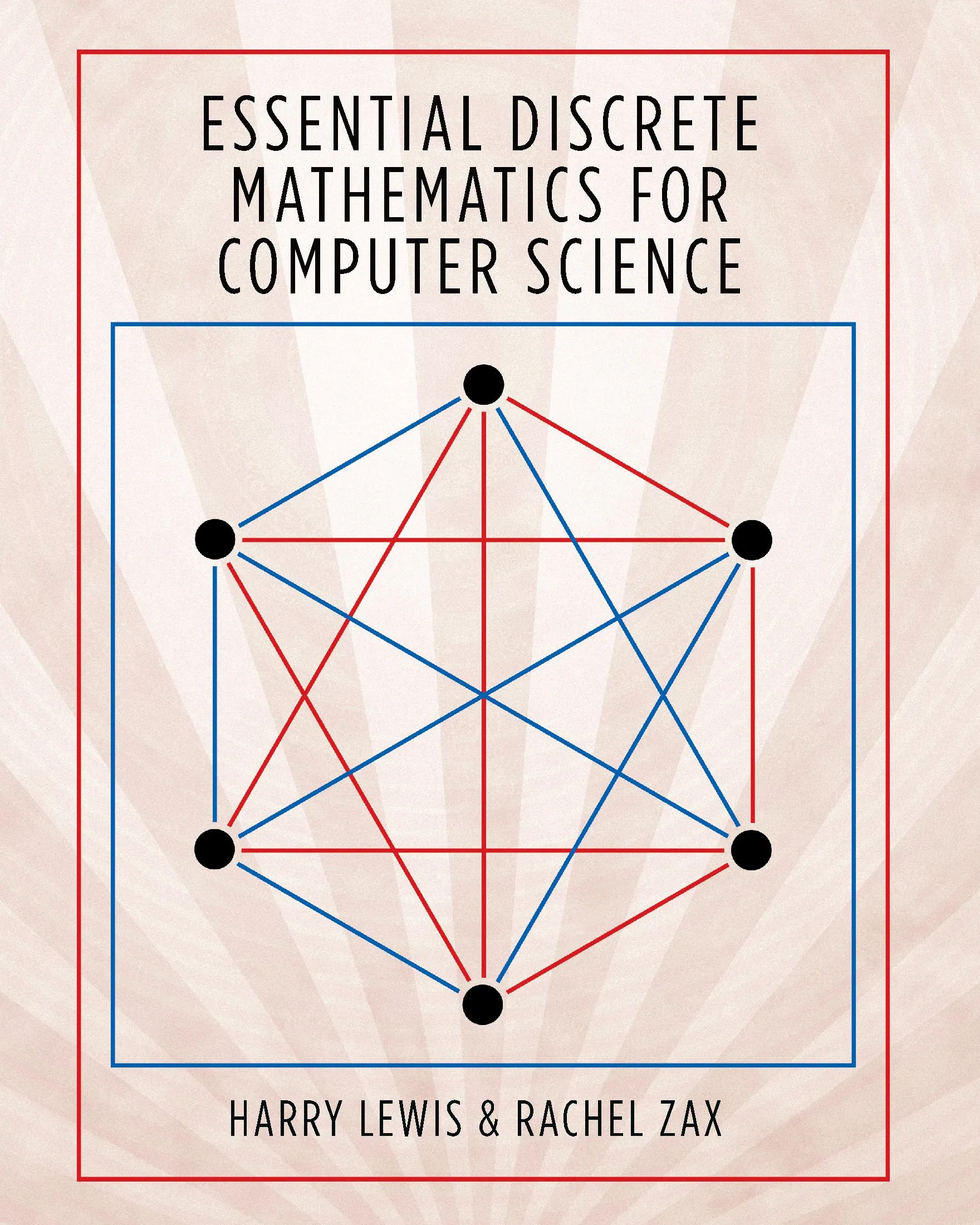#### Essential Discrete Mathematics for Computer Science

Harry Lewis

##### ebooks

Our ebooks editions are available from these online vendors:

Many of our ebooks are available through library electronic resources including these platforms:

# Essential Discrete Mathematics for Computer Science

A more intuitive approach to the mathematical foundation of computer science### ebook

ISBN:
Published:
03/19/2019
2019
Illus:
116 color + 77 b/w illus. 19 tables

Discrete mathematics is the basis of much of computer science, from algorithms and automata theory to combinatorics and graph theory. This textbook covers the discrete mathematics that every computer science student needs to learn. Guiding students quickly through thirty-one short chapters that discuss one major topic each, this flexible book can be tailored to fit the syllabi for a variety of courses.

Proven in the classroom, Essential Discrete Mathematics for Computer Science aims to teach mathematical reasoning as well as concepts and skills by stressing the art of proof. It is fully illustrated in color, and each chapter includes a concise summary as well as a set of exercises. The text requires only precalculus, and where calculus is needed, a quick summary of the basic facts is provided.

Essential Discrete Mathematics for Computer Science is the ideal introductory textbook for standard undergraduate courses, and is also suitable for high school courses, distance education for adult learners, and self-study.

• The essential introduction to discrete mathematics
• Features thirty-one short chapters, each suitable for a single class lesson
• Includes more than 300 exercises
• Almost every formula and theorem proved in full
• Breadth of content makes the book adaptable to a variety of courses
• Each chapter includes a concise summary
• Solutions manual available to instructors